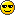9jaloud Forums

# 2018 Neco Mathematics Objective and Theory Questions and Answers | Mathematics Neco Expo

2018 Neco Mathematics Objective and Theory Questions and Answers is available ,Mathematics 2018 Neco Exam Expo, all you need to do is to follow the instructions above, get verified Mathematics Objective and Theory Questions and Answers here, question and answers are now Available. expo for Neco 2018 Mathematics, exam runs for Mathematcs objective and Theory questions and answersNeco maths portal

MATHS OBJ:
1-10: CDAAEABAEC
11-20: ACDDCDCDACu
31-40: CBEECCBDCC
41-50: DDCBCDDBBA
51-60: BCECCBBCEE

(1a)
Log10(20x - 10) - log10(x+3) = log10^5
Log10(20x-10/x+3)= log10^5
20x - 10/x + 3 = 5
Cross multiplying
20x - 10 = 5(x + 3)
5(4x - 2) = 5(x + 3)
4x - 2 = X + 3
4x - x = 3+2
3x = 5
X = 5/3 OR 1 whole no 2/3

(1b)
Let actual amount be #X
15% of #x = #600
15x/100 = 600
X = (100/15)*600
X = 100*40
X = 4,000
Actual amount = #4,000

(2a)
(X^2 Y^-3 Z)^3/4/X^-1 Y^4 Z^5
= (X^2)^3/4/X^-1 * (Y^-3)^3/4/Y^4 * Z^3/4/Z^5
= X^3/2/X^-1 * Y^-9/4/Y^4 * Z^3/4/Z^5
=X^3/2+1  * Y^-9/4-4 * Z^3/4-5
=X^5/2  * Y^-25/4 * Z^-17/4
=X^10/4 * Y^-25/4 * Z^-17/4

=(X^10/Y^25 Z^17)^1/4

(2b)
√2/k + √2 = 1/k - √2
Multiply both sides by (k+√2)(k-√2)
√2(k-√2) = k+√2
√2k-√2 = k+√2
√2k-k = 2+√2
K(√2 -1) = 2+√2
K = 2+√2/√2-1
K = -(2+√2)/1-√2
Rationalizing
K = -(2+√2) * 1+√2/1-√2
K = -(2+√2)(1+√2)/1 - 2
K = (2+√2)(1+√2)
K = 2+2√2 + √2+2
K = 4+3√2

(4)
Draw the diagram

(i) Arc length = Tita/360*2πr
= 72/360*2*22/7*14
=1/5*44*2
=88/5
=17.6cm

(ii) Perimeter of Sector = arc length +2r
=17.6+2(14)
=17.6+28
=45.6cm

(iii) Area of sector = Tita/360*πr²
=72/360*22/7*14/1*14/1
=1/5*22*2*14
=616/5
=123.2cm2

(5a)
Mode = mass with highest frequency = 35kg
Median is the 18th mass
= 40kg.

(5b)
In a tabular form

Under Masses(x kg)
30,35,40,45,50,55

Under frequency(f)
5,9,7,6,4,4
Ef = 35

Under X-A
-10, -5, 0, 5, 10, 15

Under F(X-A)
-50, -45, 0, 30, 40, 60
Ef(X - A) = 35

Mean = A + (Ef(X - A)/Ef)
= 40 + 35/35
= 40 + 1
= 41kg

(x=a+by(eqi)
when y=5 and x=19
19=a+5b(eqii)
when y=10 and x=34
34=a+10b(eqiii)
solving eqii and eqiii
a+10b=34
a+5b=19
=>5b=15
b=15/5=3
putting b=3 in eqii
19=a+5(3)
19=a+15
a=19-15
a=4

(8i)
Putting a=4 and b=3 in eqi
x=4+3y
This is the relationship between xand y

(8ii)
When y=7
x=4+3(7)
x=4+21
x=25

11a)
x+y/2 =11
x+y= 11*2
x+y= 22 ---(1)
x-y= 4 ----(11)
x+y = 22----(1)
-
x-y= 4----(11)
____________
2y = 18
y= 18/2
y=9
Substitute y=9 in equ 1
x+9=22
x=22-9
x=13
x=13, y=9
x+y= 13+9= 22
Sum of the two number

(11b)
(6x + 3) dx
(6x + 3)dx
(6x +3)^6 - (6x + 3)^1
(6 x + 3)^5
(7776x^5 + 243)
38,880x/6 + 243
6480 x^6 + 243x
9(720x^6 + 27x)

(11c)
y = x² + 5x - 3 (x = 2)
y = 2² + 5(2) - 3
y = 4 + 10 - 3
y = 14 - 3
y = 11
Gradient of the curve = 11

Monday 4th June 2018

OBJ � General Mathematics � 10:00am � 11:45am
Essay � General Mathematics � 12:00noon �2:30pm

Mathematics Neco exam will be deliver Night Before the exam day, we are not going to post answers here for free, Check our previous exam>>>  2018 Neco Geography Objective & Practical / Physical Questions and Answers | Geography Expo

Also Read>> Neco 2018 Whatsapp Exam Runs Package | Neco Exam Expo

Procedure on How To Subscribe for 2018 Neco Mathematics Objective and Theory Questions and Answers

No more Direct mobile this time, our answers delivery will be strictly via whatsapp.

Also Read>> 2018 Verified Neco Timetable

First Procedure on how to Subscribe

To subscribe for Mathematics Objective and Theory Questions and Answers send the card pin, Subject name(Mathematics Objective and Theory Questions and Answers)  and your phone number Via SMS To 08065889844

Second Procedure On How To Subscribe

Here you can send us the card pin and subject name( Mathematics Objective and Theory Questions and Answers) Directly to our whatsapp 08065889844

Mind you our  Mathematics Objective and Theory Questions and Answers is N700 MTN Card

Subscription will End 1 hour before each exam start

Note: please do not call our number, simply send message on whatsapp or using SMS

2018 Neco Mathematics Objective and Theory Questions and Answers is available ,all you need to do is to follow the instructions above, get verified Mathematics Objective and Theory Questions and Answers here, question and answers are now Available. expo for Neco 2018 Mathematics, exam runs for Mathematcs objective and Theory questions and answers

2018 Neco Mathematics Objective and Theory Questions and Answers is available ,all you need to do is to follow the instructions above, get verified Mathematics Objective and Theory Questions and Answers here, question and answers are now Available. expo for Neco 2018 Mathematics, exam runs for Mathematcs objective and Theory questions and answers
Also Read>>  2018 Neco Biology Objective and Essay Questions and Answers | Biology Expo

2018 Neco Mathematics Objective and Theory Questions and Answers is available ,all you need to do is to follow the instructions above, get verified Mathematics Objective and Theory Questions and Answers here, question and answers are now Available. expo for Neco 2018 Mathematics, exam runs for Mathematcs objective and Theory questions and answers

Last edited by Emmi on Mon Jun 04, 2018 11:58 am; edited 6 times in total
Our Subscribers have gotten their Answers
I have very less time left for the LSAT Prep but there is more than half syllabus remaining to cover up. Completing all of the topics will take up a lot of my time as studying alone is boring now because of no guidance. That’s why I’m thinking of joining a good LSAT coaching class for this purpose.
Permissions in this forum:
You can reply to topics in this forum